# Longest Common Subsequence | (DP – 25)

Problem Statement: Introduction to DP on Strings – Longest Common Subsequence

In the coming articles, we will discuss problems related to ‘Dynamic Programming on Strings’. We will discuss the problem of ‘Longest Common Subsequence’ in this article. Before proceeding further, let us understand what is the “Longest Common Subsequence”, or rather what is a “subsequence”?

A subsequence of a string is a list of characters of the string where some characters are deleted ( or not deleted at all) and they should be in the same order in the subsequence as in the original string.

For eg:

`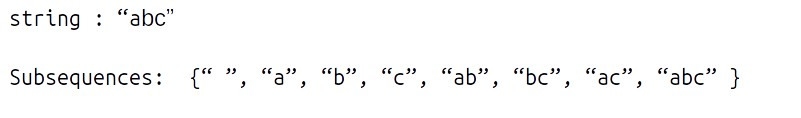`

Strings like “cab”,” bc” will not be called as a subsequence of “abc” as the characters are not coming in the same order.

Note: For a string of length n, the number of subsequences will be 2n.

Now we will look at “Longest Common Subsequence”. The longest Common Subsequence is defined for two strings. It is the common subsequence that has the greatest length.

For example:

`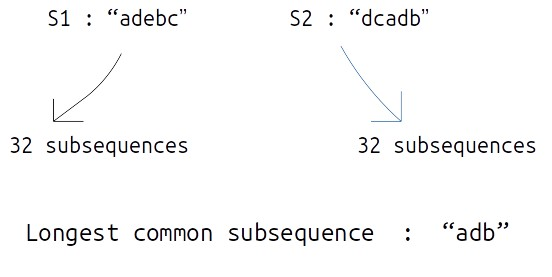`

Problem Link: Longest Common Subsequence

### Solution :

Approach 1: Using Brute Force

We are given two strings, S1, and S2 (suppose of same length n), the simplest approach will be to generate all the subsequences and store them, then manually find out the longest common subsequence.

This naive approach will give us the correct answer but to generate all the subsequences, we will require exponential ( 2n ) time. Therefore we will try some other approaches.

Approach 2: Using Dynamic Programming

We would want to try something that can give us the longest common subsequence on the way of generating all subsequences. To generate all subsequences we will use recursion and in the recursive logic we will figure out a way to solve this problem.

Steps to form the recursive solution:

We will first form the recursive solution by the three points mentioned in Dynamic Programming Introduction

Step 1: Express the problem in terms of indexes.

We are given two strings S1 and S2:

`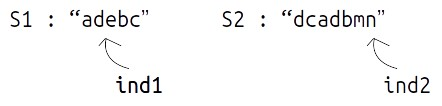`

A single variable can’t express both the strings at the same time, so we will use two variables ind1 and ind2. They mean that we are considering string S1 from index 0 ind1 and string S2 from index 0 to S2. So our recursive function will look like this:Step 2: Explore all possibilities at a given index

Intuition for Recursive Logic

In the function f(ind1,ind2), ind1 and ind2 are representing two characters from strings S1 and S2 respectively.  For example:

`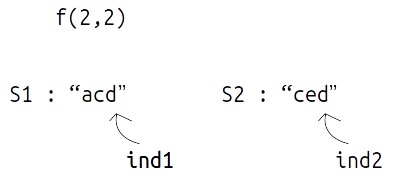`

Now, there can be two possibilities,

• if(S1[ind1] == S2[ind2]) as in the figure below. In this case this common element will represent a unit length common subsequence, so we can say that we have found one character and we can shrink both the strings by 1 to find the longest common subsequence in the remaining pair of strings.
`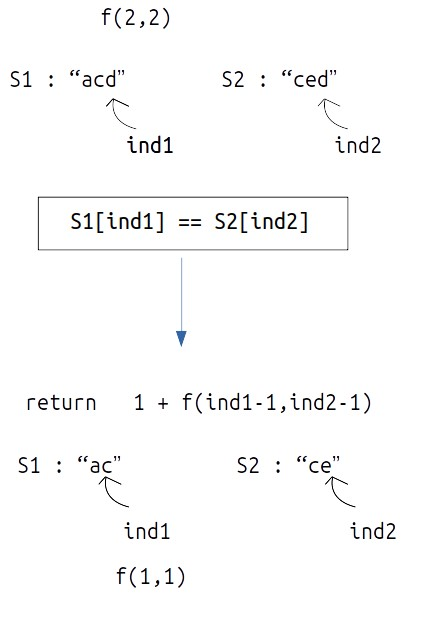`
• if(S1[ind1] != S2[ind2]) as in the figure given below. In this case we know that the current characters represented by ind1 and ind 2 will be different. So, we need to compare the ind1 character with shrunk S2 and ind2 with shrunk S1. But how do we make this comparison ?  If we make a single recursive call as we did above to f(ind1-1,ind2-1), we may lose some characters of the subsequence. Therefore we make two recursive calls: one to f(ind1,ind2-1) (shrinking only S1) and one to f(ind1-1,ind2) (shrinking only S2). Then when we return max of both the calls.
`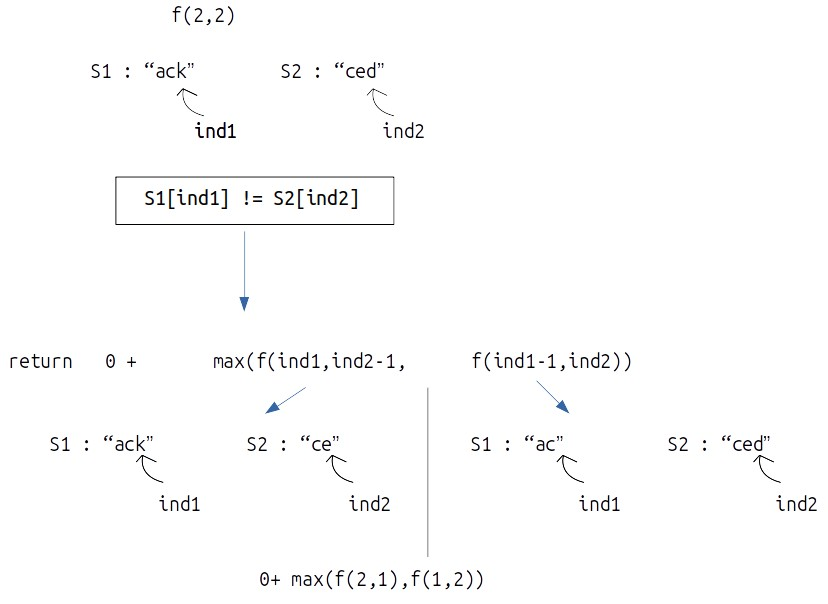`

Step 3:  Return the maximum of the choices

In the first case, we have only one choice but in the second case we have two choices, as we have to return the longest common subsequences, we will return the maximum of both the choices in the second case.

Base Case:

For a case like this:

`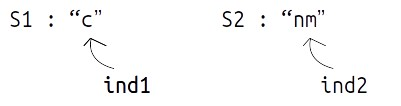`

As S1[ind1] != S2[ind2]

We will make a call to f(0-1,1), i.e f(-1,1) but a negative index simply means that there are no more indexes to be explored, so we simply return 0. Same is the case when S1[ind1]==S2[ind2]

• If (ind1<0 || ind2<0) return 0.

The final pseudocode after steps 1, 2, and 3: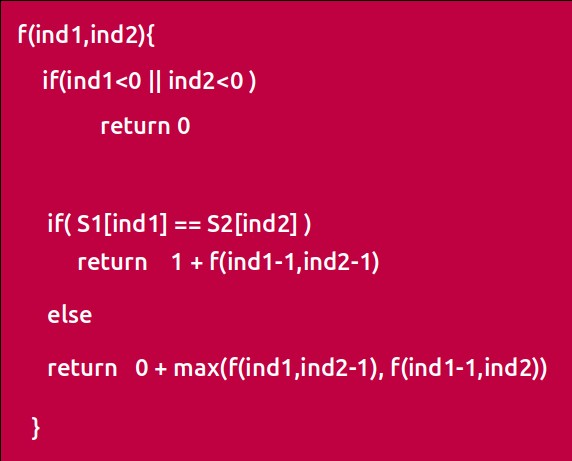Recursive Tree

We will dry run this example: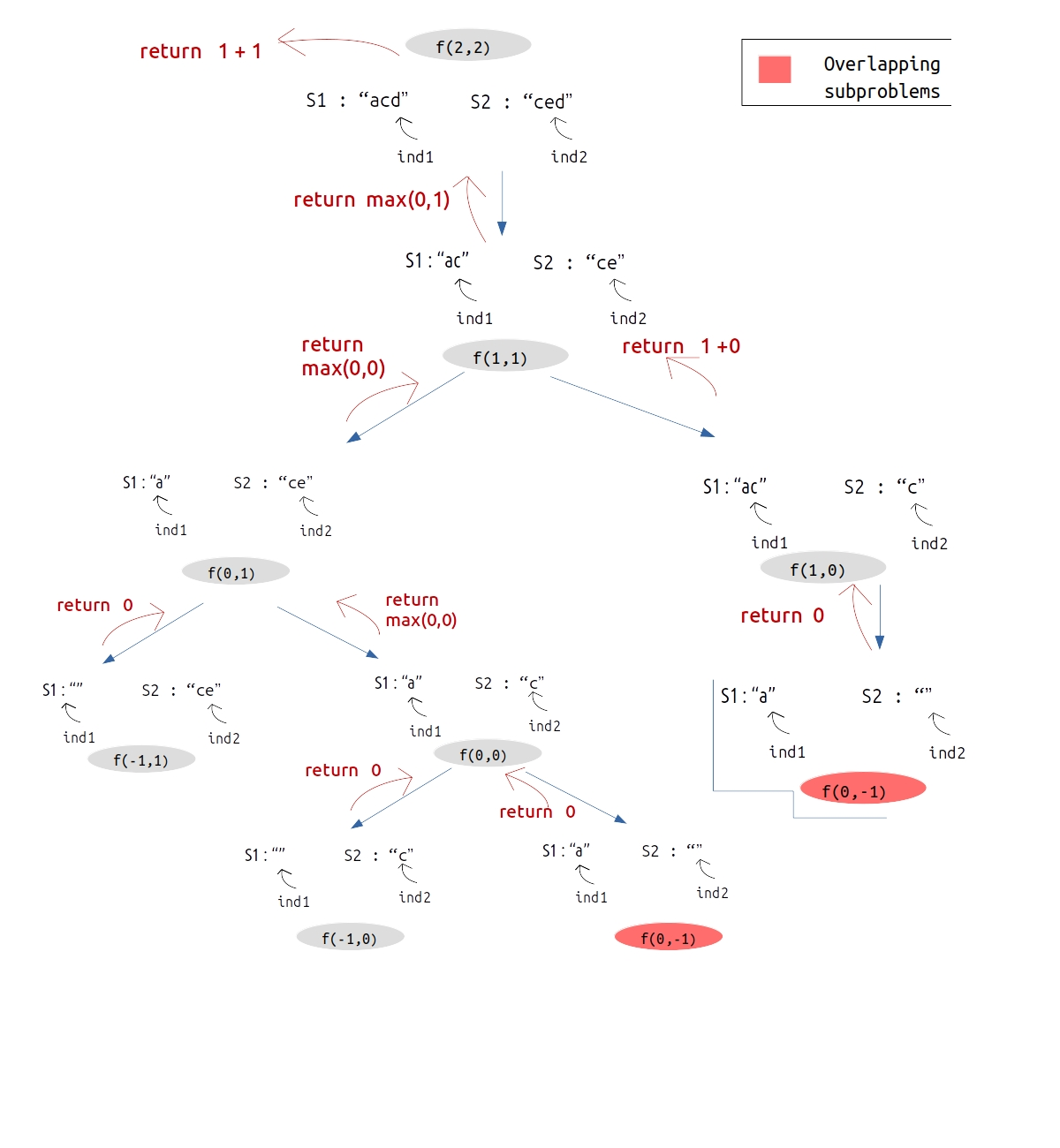Steps to memoize a recursive solution:

As we see there are overlapping subproblems in the recursive tree, we can memorize the recursive code to reduce the time complexity.

1. Create a dp array of size [N][M] where N and M are lengths of S1 and S2 respectively. It will store all the possible different states that our recursive function will take.
2. We initialize the dp array to -1.
3. Whenever we want to find the answer of particular parameters (say f(ind1,ind2)), we first check whether the answer is already calculated using the dp array(i.e dp[ind][ind2]!= -1 ). If yes, simply return the value from the dp array.
4. If not, then we are finding the answer for the given value for the first time, we will use the recursive relation as usual but before returning from the function, we will set dp[ind][ind2] to the solution we get.

Code:

## C++ Code

``````#include <bits/stdc++.h>

using namespace std;

int lcsUtil(string& s1, string& s2, int ind1, int ind2,  vector<vector<int>>& dp){

if(ind1<0 || ind2<0)
return 0;

if(dp[ind1][ind2]!=-1)
return dp[ind1][ind2];

if(s1[ind1] == s2[ind2])
return dp[ind1][ind2] = 1 + lcsUtil(s1,s2,ind1-1,ind2-1,dp);

else
return dp[ind1][ind2] = 0 + max(lcsUtil(s1,s2,ind1,ind2-1,dp),lcsUtil
(s1,s2,ind1-1,ind2,dp));

}

int lcs(string s1, string s2) {

int n=s1.size();
int m=s2.size();

vector<vector<int>> dp(n,vector<int>(m,-1));
return lcsUtil(s1,s2,n-1,m-1,dp);
}

int main() {

string s1= "acd";
string s2= "ced";

cout<<"The Length of Longest Common Subsequence is "<<lcs(s1,s2);
}
``````

Output: The Length of Longest Common Subsequence is 2

Time Complexity: O(N*M)

Reason: There are N*M states therefore at max ‘N*M’ new problems will be solved.

Space Complexity: O(N*M) + O(N+M)

Reason: We are using an auxiliary recursion stack space(O(N+M)) (see the recursive tree, in the worst case, we will go till N+M calls at a time) and a 2D array ( O(N*M)).

## Java Code

``````import java.util.*;

class TUF{
static int lcsUtil(String s1, String s2, int ind1, int ind2, int[][] dp){

if(ind1<0 || ind2<0)
return 0;

if(dp[ind1][ind2]!=-1)
return dp[ind1][ind2];

if(s1.charAt(ind1) == s2.charAt(ind2))
return dp[ind1][ind2] = 1 + lcsUtil(s1,s2,ind1-1,ind2-1,dp);

else
return dp[ind1][ind2] = 0 + Math.max(lcsUtil(s1,s2,ind1,ind2-1,dp),
lcsUtil(s1,s2,ind1-1,ind2,dp));

}

static int lcs(String s1, String s2) {

int n=s1.length();
int m=s2.length();

int dp[][]=new int[n][m];
for(int rows[]: dp)
Arrays.fill(rows,-1);
return lcsUtil(s1,s2,n-1,m-1,dp);
}

public static void main(String args[]) {

String s1= "acd";
String s2= "ced";

System.out.println("The Length of Longest Common Subsequence is "+lcs(s1,s2));
}
}
``````

Output: The Length of Longest Common Subsequence is 2

Time Complexity: O(N*M)

Reason: There are N*M states therefore at max ‘N*M’ new problems will be solved.

Space Complexity: O(N*M) + O(N+M)

Reason: We are using an auxiliary recursion stack space(O(N+M)) (see the recursive tree, in the worst case, we will go till N+M calls at a time) and a 2D array ( O(N*M)).

### Steps to convert Recursive Solution to Tabulation one.

To convert the memoization approach to a tabulation one, create a dp array with the same size as done in memoization.

### Initialization: Shifting of indexes

In the recursive logic, we set the base case to if(ind1<0 || ind2<0) but we can’t set the dp array’s index to -1. Therefore a hack for this issue is to shift every index by 1 towards the right.

`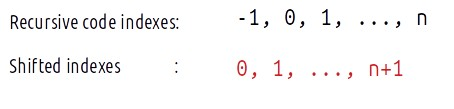`
• Therefore, now the base case will be if(ind1==0 || ind2==0).
• Similarly, we will implement the recursive code by keeping in mind the shifting of indexes, therefore S1[ind1] will be converted to S1[ind1-1]. Same for others.
• At last we will print dp[N][M] as our answer.

Code:

## C++ Code

``````#include <bits/stdc++.h>

using namespace std;

int lcs(string s1, string s2) {

int n=s1.size();
int m=s2.size();

vector<vector<int>> dp(n+1,vector<int>(m+1,-1));
for(int i=0;i<=n;i++){
dp[i] = 0;
}
for(int i=0;i<=m;i++){
dp[i] = 0;
}

for(int ind1=1;ind1<=n;ind1++){
for(int ind2=1;ind2<=m;ind2++){
if(s1[ind1-1]==s2[ind2-1])
dp[ind1][ind2] = 1 + dp[ind1-1][ind2-1];
else
dp[ind1][ind2] = 0 + max(dp[ind1-1][ind2],dp[ind1][ind2-1]);
}
}

return dp[n][m];
}

int main() {

string s1= "acd";
string s2= "ced";

cout<<"The Length of Longest Common Subsequence is "<<lcs(s1,s2);
}
``````

Output:

The Length of Longest Common Subsequence is 2

Time Complexity: O(N*M)

Reason: There are two nested loops

Space Complexity: O(N*M)

Reason: We are using an external array of size ‘N*M)’. Stack Space is eliminated.

## Java Code

``````import java.util.*;

class TUF{
static int lcs(String s1, String s2) {

int n=s1.length();
int m=s2.length();

int dp[][]=new int[n+1][m+1];
for(int rows[]: dp)
Arrays.fill(rows,-1);

for(int i=0;i<=n;i++){
dp[i] = 0;
}
for(int i=0;i<=m;i++){
dp[i] = 0;
}

for(int ind1=1;ind1<=n;ind1++){
for(int ind2=1;ind2<=m;ind2++){
if(s1.charAt(ind1-1)==s2.charAt(ind2-1))
dp[ind1][ind2] = 1 + dp[ind1-1][ind2-1];
else
dp[ind1][ind2] = 0 + Math.max(dp[ind1-1][ind2],dp[ind1][ind2-1]);
}
}

return dp[n][m];
}

public static void main(String args[]) {

String s1= "acd";
String s2= "ced";

System.out.println("The Length of Longest Common Subsequence is "+lcs(s1,s2));
}
}
``````

Output:

The Length of Longest Common Subsequence is 2

Time Complexity: O(N*M)

Reason: There are two nested loops

Space Complexity: O(N*M)

Reason: We are using an external array of size ‘N*M)’. Stack Space is eliminated.

### Part 3: Space Optimization

If we closely we are using two rows: dp[ind1-1][ ], dp[ind][ ],

So we are not required to contain an entire array, we can simply have two rows prev and cur where prev corresponds to dp[ind-1] and cur to dp[ind].

After declaring prev and cur, replace dp[ind-1] to prev and dp[ind] with cur and after the inner loop executes, we will set prev = cur, so that the cur row can serve as prev for the next index.

Code:

## C++Code

``````#include <bits/stdc++.h>

using namespace std;

int lcs(string s1, string s2) {

int n=s1.size();
int m=s2.size();

vector<int> prev(m+1,0), cur(m+1,0);

// Base Case is covered as we have initialized the prev and cur to 0.

for(int ind1=1;ind1<=n;ind1++){
for(int ind2=1;ind2<=m;ind2++){
if(s1[ind1-1]==s2[ind2-1])
cur[ind2] = 1 + prev[ind2-1];
else
cur[ind2] = 0 + max(prev[ind2],cur[ind2-1]);
}
prev= cur;
}

return prev[m];
}

int main() {

string s1= "acd";
string s2= "ced";

cout<<"The Length of Longest Common Subsequence is "<<lcs(s1,s2);
}
``````

Output:

The Maximum price generated is 12

Time Complexity: O(N*M)

Reason: There are two nested loops.

Space Complexity: O(M)

Reason: We are using an external array of size ‘M+1’ to store only two rows.

## Java Code

``````import java.util.*;

class TUF{
static int lcs(String s1, String s2) {

int n=s1.length();
int m=s2.length();

// Base Case is covered as we have initialized the prev and cur to 0.
int prev[]=new int[m+1];
int cur[]=new int[m+1];
for(int ind1=1;ind1<=n;ind1++){
for(int ind2=1;ind2<=m;ind2++){
if(s1.charAt(ind1-1)==s2.charAt(ind2-1))
cur[ind2] = 1 + prev[ind2-1];
else
cur[ind2] = 0 + Math.max(prev[ind2],cur[ind2-1]);
}
prev=(int[])(cur.clone());
}

return prev[m];
}

public static void main(String args[]) {

String s1= "acd";
String s2= "ced";

System.out.println("The Length of Longest Common Subsequence is "+lcs(s1,s2));
}
}
``````

Output:

The Maximum price generated is 12

Time Complexity: O(N*M)

Reason: There are two nested loops.

Space Complexity: O(M)

Reason: We are using an external array of size ‘M+1’ to store only two rows.

Special thanks to Anshuman Sharma for contributing to this article on takeUforward. If you also wish to share your knowledge with the takeUforward fam, please check out this article